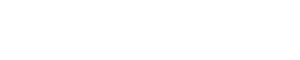# Mechanics of Machines-1

#### Course Topics

• Introduction
• Turning Moment Diagram and Flywheel
• Functions of Flywheel
• Crank Effort Diagram
• Fluctuation of Energy and Speed
• Effect of centrifugal force
• Kinematics and Link Mechanisms
• Relative motion between bodies
• Instantaneous centre method
• Rubbing velocities at pin joints
• Graphical construction of relative velocity and acceleration
• Effect of friction
• CAMS
• Types of cams and followers
• Specified motion of followers
• graphical construction of Cam profile
• In line cams and Off center cams
• SPUR GEARING
• Various definition of Gear parameters
• Length of path of contact
• Law of gearing
• Length of path of contact
• Length of arc of contact
• Velocity of Sliding of Gear teeth
• Interference in mating gears
• Gear ratio
• Center distance of mating gears
• Toothed gearing
• Types of gears
• Power transmission by Gear trains
• Types of gear trains
• Torque on gear trains
• Methods of avoiding interference
• Gyroscopic couple
• Vector representation of torque and angular momentum
• Motion involving steady precession
• Application to Ship’s stabilization
• Reaction on gearbox bearings

## Introduction to Mechanics of MACHINES.

Theory of Machines may be defined as that branch of Engineering-science, which deals with the study of relative motion between the various parts of a machine, and forces which act on them.# The Theory of Machines may be sub-divided into the following four branches :

The study of machines or simple mechanisms , and the analysis of these parts connected together with links and pivots and levers is the theory of it.
We could say that the geometrical analysis which includes the locating of the centre of gravity of the whole mechanism is what it is.  It's is also the study of the effects of forces or loads or and torque on these objects through bending moment and force diagrams .

1. Kinematics. It is that branch of Theory of Machines which deals with the relative motion between the various parts of the machines.
2. Dynamics. It is that branch of Theory of Machines which deals with the forces and their effects, while acting upon the machine parts in motion.
3. Kinetics. It is that branch of Theory of Machines which deals with the inertia forces which arise from the combined effect of the mass and motion of the machine parts.
4. Statics. It is that branch of Theory of Machines which deals with the forces and their effects while the ma- chine parts are at rest. The mass of the parts is assumed to be negligible.### Mechanics of Machines

The subject Mechanics of Machines may be defined as that branch of Engineering science which deals with the study of relative motion between the various parts of a machine and forces which act on these parts due to constrained motion. The study of relative motion alone is referred to as Kinematics while that of forces acting on these parts is called dynamics.

Kinematics

Velocity: This is defined as the rate of change of displacement linear and angular of a body with respect to the time. Velocity is a vector quantity, to specify it completely the magnitude, direction and sense must be known. Acceleration: The acceleration of a body is the rate of change of its velocity linear or angular with respect to time. A body accelerates if there is a change in either the magnitude, direction or sense of its velocity and can thus accelerates without change in speed, as in the case of a body moving in a circular path with uniform speed.

Displacement: Displacement is defined as the distance moved by a body with respect to a certain fixed point. The displacement may be along a straight or a curved path. Equations of Uniformly accelerated Motion.

Let a body having linear motion accelerates uniformly from an initial velocity u to a final velocity v in time t; let the acceleration be a and the distance from the initial position be s. Then V= u + at

S = ut + at2 ,

V2 = u2 + 2as

The corresponding equations for angular motion are:

ὠ =ꭃ + at

ꝋ = ꭃ t + ½ a t 2 ,

then its angular velocity, ὠ =  2πN/60 rad/ s.